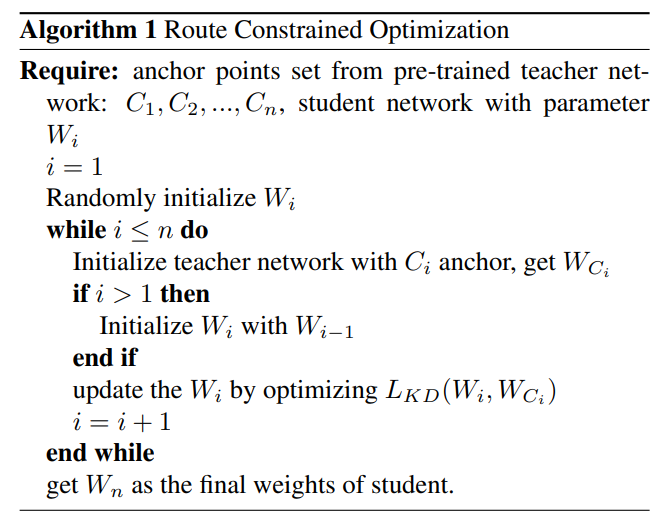# Route Constrained Optimization using KD_Lib¶

Paper

• The route constrained optimization algorithm considers knowledge distillation from the perspective of curriculum learning by routing
• Instead of supervising the student model with a converged teacher model, it is supervised with some anchor points selected from the route in parameter space that the teacher model passed by
• This has been demonstrated to greatly reduce the lower bound of congruence loss for knowledge distillation, hint and mimicking learningTo use RCO with the the student mimicking the teacher’s trajectory at an interval of 5 epochs -

import torch
import torch.nn as nn
import torch.optim as optim
from torchvision import datasets, transforms
from KD_Lib.KD import RCO

# Define datasets, dataloaders, models and optimizers

datasets.MNIST(
"mnist_data",
train=True,
transform=transforms.Compose(
[transforms.ToTensor(), transforms.Normalize((0.1307,), (0.3081,))]
),
),
batch_size=32,
shuffle=True,
)

datasets.MNIST(
"mnist_data",
train=False,
transform=transforms.Compose(
[transforms.ToTensor(), transforms.Normalize((0.1307,), (0.3081,))]
),
),
batch_size=32,
shuffle=True,
)

# Set device to be trained on

device = torch.device("cuda:0" if torch.cuda.is_available() else "cpu")

# Define student and teacher models

# Define optimizers

teacher_optimizer = optim.SGD(teacher_model.parameters(), lr=0.01)
student_optimizer = optim.SGD(student_model.parameters(), lr=0.01)

# Train using KD_Lib